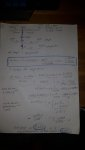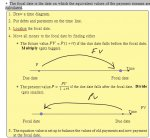I seem to make a small mistake (picture w/ calculation): "You borrowed some money..."

Niek89

New member
I seem to make a small mistake (picture w/ calculation): "You borrowed some money..."

1. You borrowed some money. Today, you were supposed to pay $12,000 and one year from now pay$7,250. Unfortunately, you cannot afford to make the $12,000 payment that is due today. You arrange to pay off both debts by making a payment 5 months from now and a second payment 1 years from now. You will be charged 8% simple interest. 1. The first payment will be$3,000 larger than the second payment. Find the size of each payment. Use 5 months as the focal date.

The correct answer: First: $11,502.43, Second:$8,502.43
My answer: i am close but still going wrong?HallsofIvy

Elite Member
I can't read your attachment but this is how I would have done this problem:

At the present moment you owe $12,000+ 7,250=$19,250. In 5 months, at an 8% interest rate, you will have accumulated (19,250)(0.08)(5/12)= $641.67 in interest. Let x be the amount you pay after the first 5 months. You now owe 19,250+ 641.67- x=$19891.67- x. The interest on that, over the next 7 months will be (19891.67- x)(0.08)(7/12)= $928.28- 0.047x. You now owe$19891.67- x+ 928.28- 0.047x= 20819.95- 1.047x= x+ 3000. That gives 2.047x= 17819.95.

x= 17819.95/2.047= $8705.40. You must pay$8705.40 after the first 5 months and $8705.40+ 3000=$11705.40 at the end of the year. That is a total of $20410.80, the original$19,250 and $1160.80 in interest. Niek89 New member I can't read your attachment but this is how I would have done this problem: At the present moment you owe$12,000+ 7,250= $19,250. In 5 months, at an 8% interest rate, you will have accumulated (19,250)(0.08)(5/12)=$641.67 in interest. Let x be the amount you pay after the first 5 months. You now owe 19,250+ 641.67- x= $19891.67- x. The interest on that, over the next 7 months will be (19891.67- x)(0.08)(7/12)=$928.28- 0.047x. You now owe $19891.67- x+ 928.28- 0.047x= 20819.95- 1.047x= x+ 3000. That gives 2.047x= 17819.95. x= 17819.95/2.047=$8705.40.

You must pay $8705.40 after the first 5 months and$8705.40+ 3000= $11705.40 at the end of the year. That is a total of$20410.80, the original $19,250 and$1160.80 in interest.
If the question says "one year from now pay $7,250" Do i need to pay straight 8% interest over it. or only if i go past that 1 year. (just like that 12000, that i could not pay now, so now i start to calculate interest?). I thought i had to do something with the 5 months focal date that i cant take the 7250 as full year interest? Also it says the first payment is 3000 LARGER than the second one. i think that should change a bit of the outcome? Denis Senior Member You borrowed some money. Today, you were supposed to pay$12,000 and one year from now pay $7,250. You cannot afford to make the$12,000 payment that is due today.
You arrange to pay off both debts by making a payment 5 months from now,
and a second payment 1 year from now.
You will be charged 8% simple interest.
The first payment will be $3,000 larger than the second payment. Find the size of each payment. The correct answer: First:$11,502.43, Second: \$8,502.43
I'm assuming there is absolutely no compounding of interest at any time.

Then, using the 2 given payments, we can "see what happens":

a = amount refinanced today (month #0)
r = monthly interest rate = .08/12

a + 5ra + 7r(a - 11502.43) = 11502.43 + 8502.43
Solve for a: a = 19020.04

So this is "the picture":
Code:
MONTH       INTEREST      PAYMENT    BALANCE
0                                  19020.04

5  [634.00]           -11502.43     7517.61

12  [350.82] 984.82     -8502.43         .00
Hope this helps you locate your small discrepancy.
I presume it may have to do with compound interest:
interest charged on the 5 month's interest of 634.00.

Niek89

New member
Thanks, this looks good. But i need to calculate the payments, so i cannot use them.
Can you please make the same calculation with "a = amount refinanced today (month #0)" as known parameter?

I am wondering what is my "a"?
In the example I have 12.000 due today and 7.250 due over 1 year... (with 8% simple interest)
Is my "a" 12.000 + 7.250/(1+0.08)?

Thanks

Denis

Senior Member
Thanks, this looks good. But i need to calculate the payments, so i cannot use them. 
Can you please make the same calculation with
a = amount refinanced today (month #0)as known parameter?

I am wondering what is my "a"?
In the example I have 12.000 due today and 7.250 due over 1 year... (with 8% simple interest)
Is my "a" 12.000 + 7.250/(1+0.08)? 
 I realise that. As I indicated, I did it that way to "see what the end product"
looks like...could possibly help figure our what "simple interest" really means.

 Correct. Owing today is 12000.00 + 7250/1.08 = ~18713
BUT that will not lead to given answers.
Some interest must compound at that point to result in the ~19020
amount that I started with...that's where the problem is;
interest MUST have been added, but I can't tell HOW:
perhaps has to do with "focal date" that you quote?
NO idea what that means...

In other words, the amount owing today MUST be ~19020
IF the 2 given payments are required AND there is absolutely
NO interest compounding.

Are you able to get the method used by your teacher
to obtain these 2 payments? If so, come back and
post it: I'm curious!!

Last edited:

Niek89

New member
 I realise that. As I indicated, I did it that way to "see what the end product"
looks like...could possibly help figure our what "simple interest" really means.

 Correct. Owing today is 12000.00 + 7250/1.08 = ~18713
BUT that will not lead to given answers.
Some interest must compound at that point to result in the ~19020
amount that I started with...that's where the problem is;
interest MUST have been added, but I can't tell HOW:
perhaps has to do with "focal sate" that you quote?
NO idea what that means...
Not sure if u can see the picture well (if not let me know), but this is what my book explains:Denis

Senior Member
Whoops...didn't see your post when I edited my previous post...

Easy enough to see what focal date means according to your book:
however, I can't see what it means with your problem:
why calculate what's owing as a single payment at end of 5th month?

Denis

Senior Member
I looked at your work (not easy, your handwriting is worse than mine!)
and noticed that by using focal date, you discounted the amount due
at end of year by 7 months, which results in your payment calculation
of 11352 and 8352, therefore assuming that the loan is paid in full
at end of month 5...that makes no sense...
Your payments are lower than the given payments of 11502 and 8502
because of this, since you're not charging interest for 7 months!

AGAIN, this focal date garbage is a complete mystery...
I hereby resign!

Denis

Senior Member
The "mystery" deepens...

We both agree that the balance owing at beginning of year
is 12000 + 7250/1.08 = 18712.96
a = 18712.96
u = 1.08
v = 1.03333...
p = ?

(3000 + p)/u + p/v = a
solve for p: p = 8348.74 ..... quite close to your 8352 !

Was this problem made up by your teacher or obtained from a math book?

Are you able to get the method used to obtain the given 11502 and 8502?

If so, when you do, could you post it here...or PM it to me? Thanks...